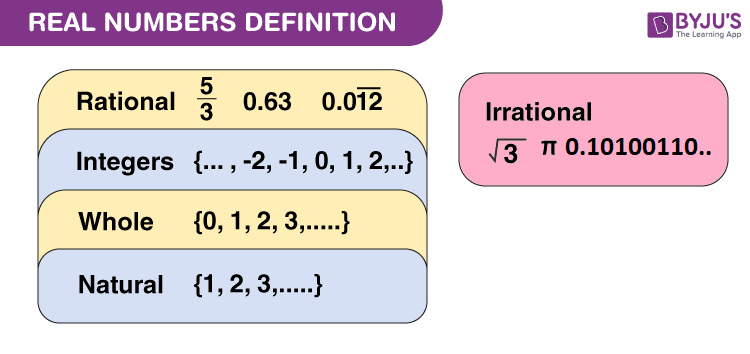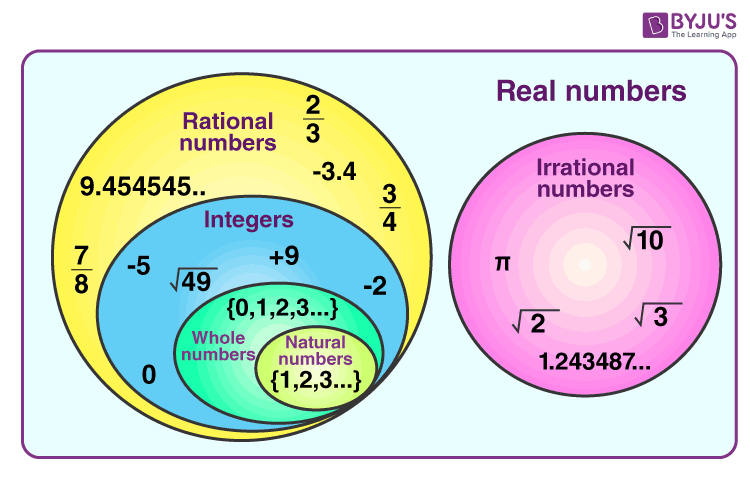# Subsets of Set of Real Numbers

Before getting started about subsets of real numbers, let’s have a look at the brief introduction of what are real numbers and what are subsets. These definitions are useful in determining the subsets of real numbers easily. In this article, you will learn what subsets are there for a set of real numbers, and how to represent them using proper notations.

What are Real numbers?

Real numbers are defined as the collection of all rational numbers and irrational numbers, denoted by R. Therefore, a real number is either rational or irrational.

The set of real numbers is: R = {…-3, -√2, -½, 0, 1, ⅘, 16,….}What is a subset?

The mathematical definition of a subset is given below:

A set A is a subset of a set B if every element of A is also an element of B. In other words, A ⊂ B if whenever a ∈ A, then a ∈ B. It is often convenient to use the symbol “⇒” which means implies. Using this symbol, we can also write the definition of the subset as,

A ⊂ B if a ∈ A ⇒ a ∈ B

## What are the Subsets of Real Numbers?

There are many important subsets of R and they are:

• The set of natural numbers N = {1, 2, 3, 4, 5, . . .}
• The set of integers Z = {. . ., –3, –2, –1, 0, 1, 2, 3, . . .}
• The set of rational numbers Q = {x: x = a/b; a, b ∈ Z and b ≠ 0}
• The set of irrational numbers, denoted by T, is composed of all other real numbers.

Thus, T = {x : x ∈ R and x ∉ Q}, i.e., all real numbers that are not rational.

Some of the irrational numbers include √2, √3, √5, and π, etc.

Some of the apparent relations among these subsets are:

N ⊂ Z ⊂ Q, Q ⊂ R, T ⊂ R, N ⊄ T.

As real numbers consist of rational numbers and irrational numbers, we can say that integers, whole numbers and natural numbers are also the subsets of real numbers. This relation can also be understood from the below figure.Thus, we can conclude the following statements.

• The set of natural numbers is the subset of whole numbers.
• The set of natural and whole numbers are the subsets of Integers.
• Integers, whole numbers, natural numbers are the subsets of Rational numbers.
• Rational numbers (including integers, whole numbers, natural numbers) and irrational numbers are the subsets of real numbers.